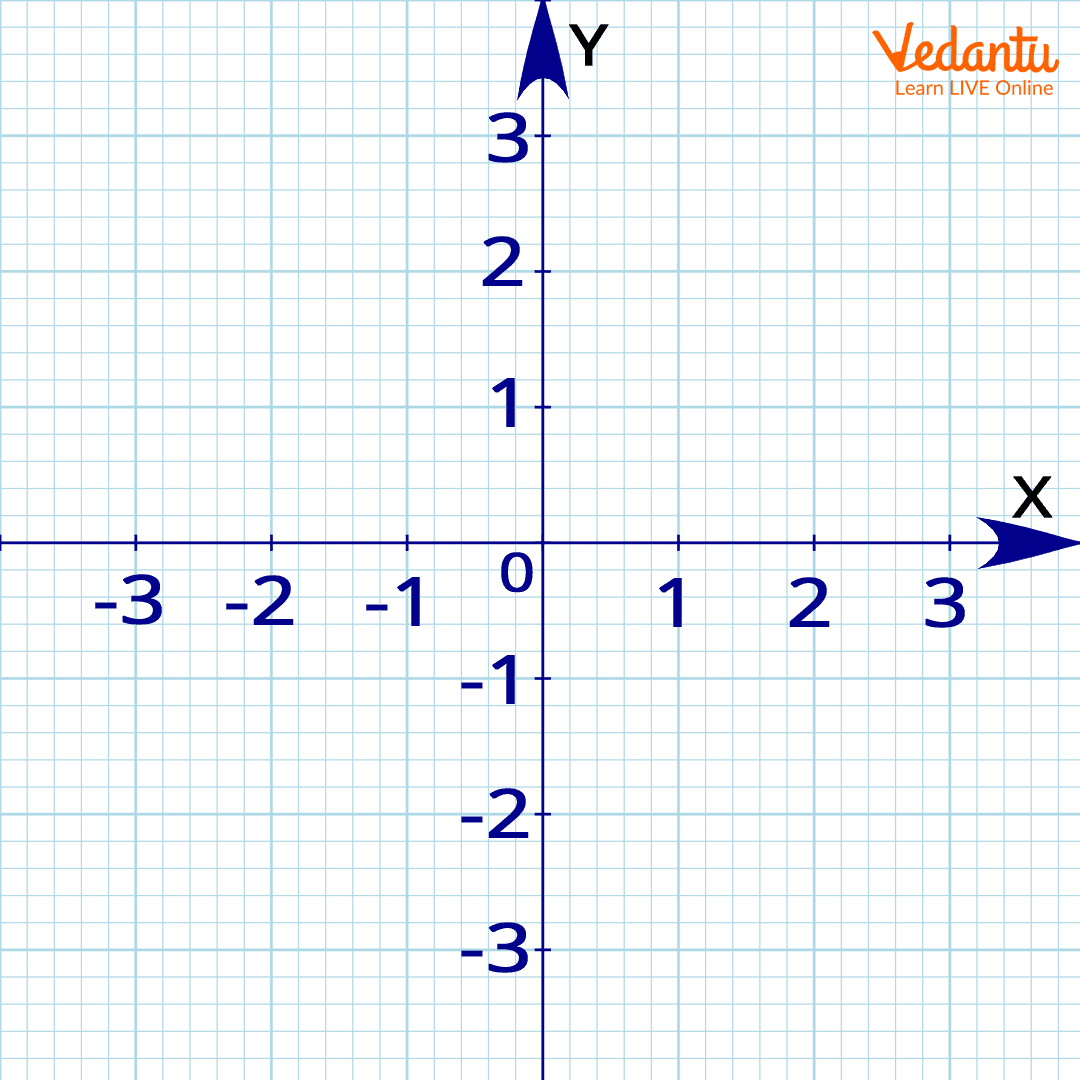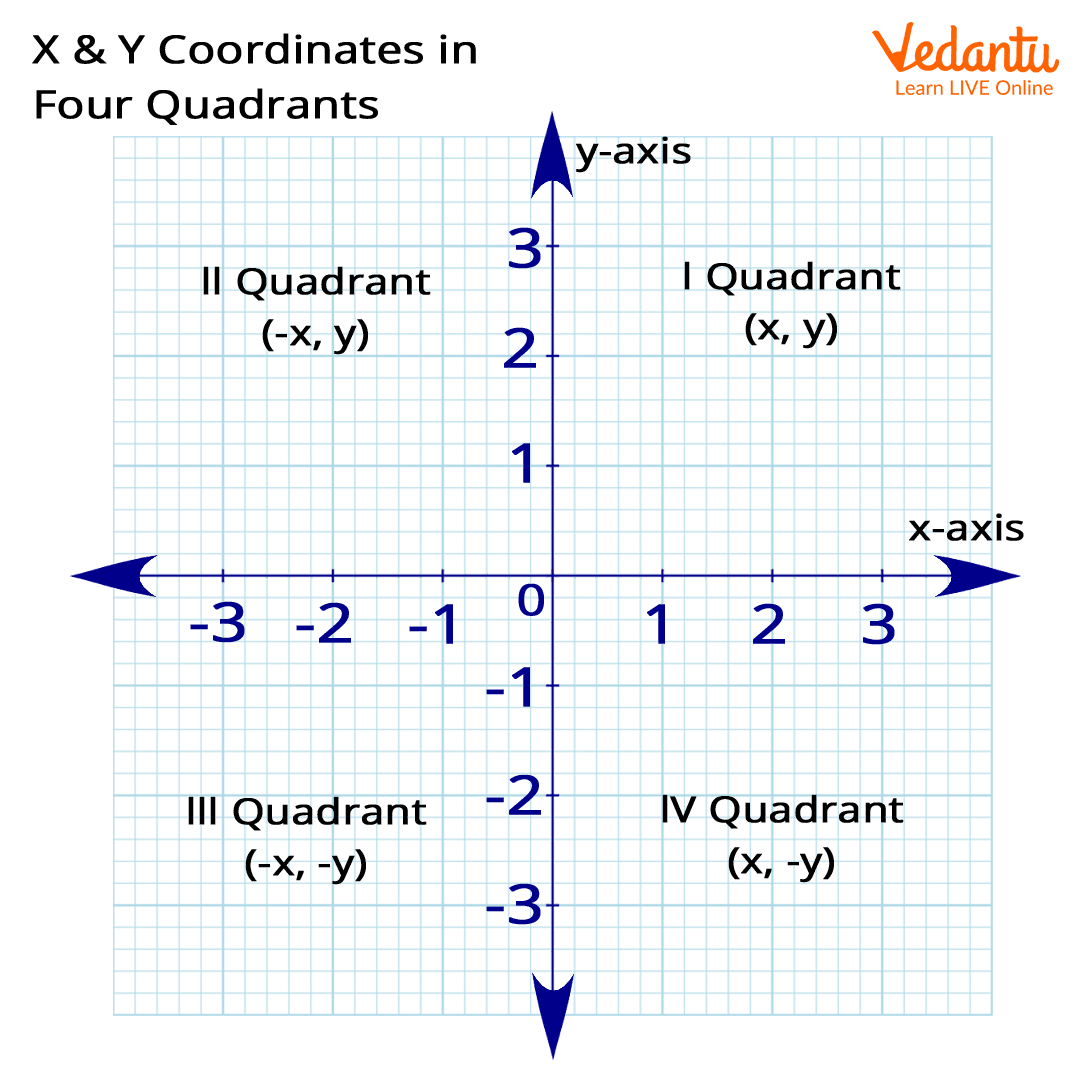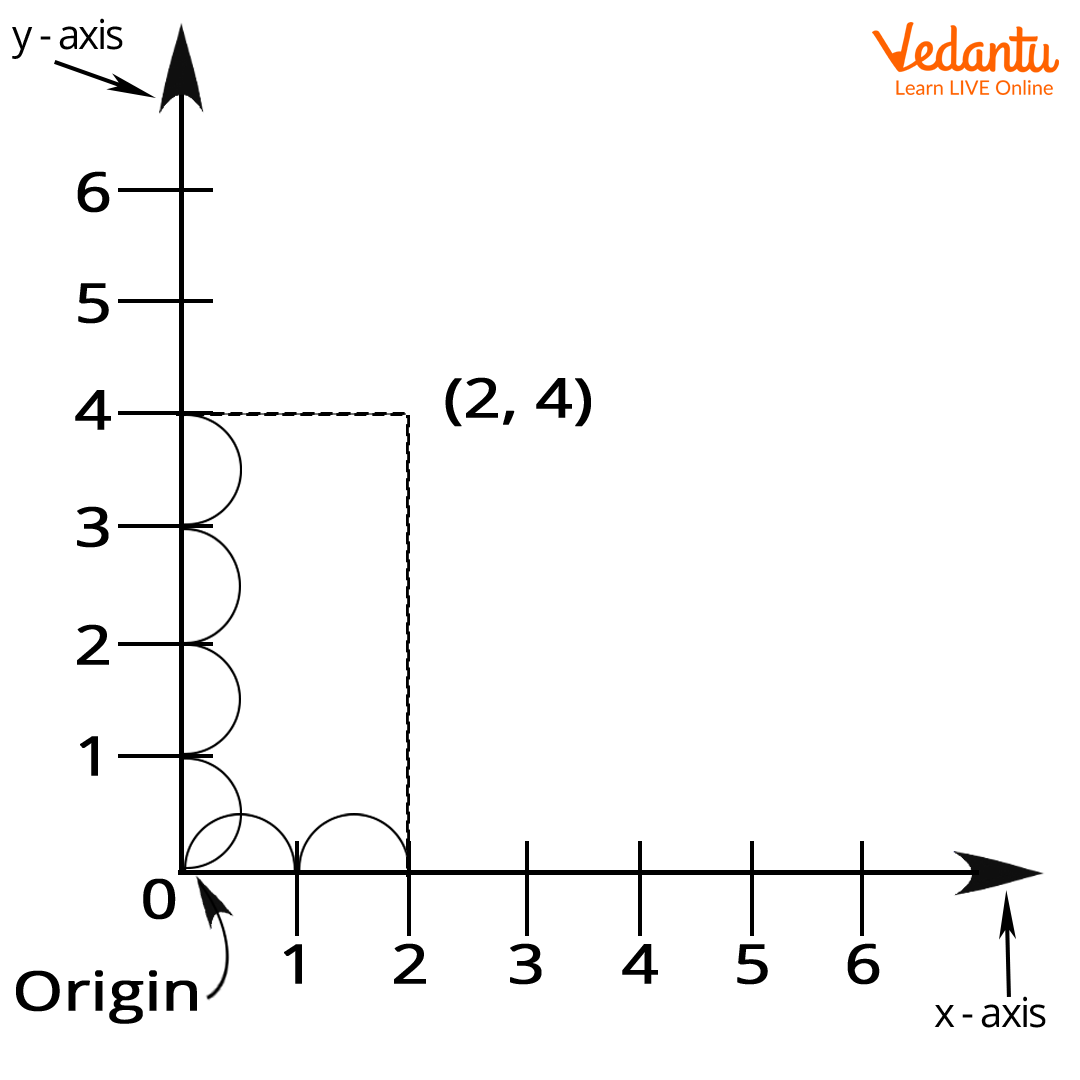Courses
Courses for Kids
Free study material
Free LIVE classes
More

# X-Coordinate - Definition and ExamplesLIVE
Join Vedantu’s FREE Mastercalss

## What is an X-coordinate?

When you enter your room, have you seen your door beam getting support from the floor or noticed that this beam and the floor are in a straight line? Do you know what they are? Well, the straight standing line is vertical and we call it a y-axis or a vertical axis, while the floor supporting this beam also has a straight line lying horizontally, thus, we call it the horizontal axis or the x-axis.

Now, these lines meet at a common point and this point always stands at the ‘0’ mark, we call this ‘0’ the origin. So, when we try to understand all these x-axis and y-axis, and the origin, we use graph paper and take some points and mark them on the x and the y-axis. So, when we mark a point having a position (x,y), we call the x and y points the coordinates of that mark.

Here, we will be focusing on the x-coordinate and how to mark it on the graph.

### What are X and Y Coordinates?

X and Y coordinates are addresses that help us to locate a point in two-dimensional space having two axes. Any point in the coordinate plane (position plane) is referred to by a point (x, y), where the x value is the position of the point with reference to the x-axis, and the y-axis value is the position of the point with reference to the y-axis.

The x and y coordinates of a point have positive or negative values that depend on the location of the point in the different quadrants.

Now, let us look at these two important terms relating to x and y coordinates.

Abscissa: It is the x value in the point (x, y), and is the distance of this point along the x-axis, from the origin.

Ordinate: It is the y value in the coordinate (x, y), and is the perpendicular distance of the point from the x-axis. Also, we describe it as the distance of this point along the y-axis, from the origin.

### How do we Mark X-coordinate on Graph?

Now, we will see a graph having an x and the y-axis along with the origin.

A graph has four quadrants just like when you fold a paper to form a square and when you unfold it, it forms two lines as you can see below:x and y coordinates on a graph

Here, the x-axis has points mentioned in blue colour, while the red ones are marked on the y-axis. 0 is the origin and on the x-axis, the right of the origin has positive values from 1 to 5, while to its left, values are negative starting from - 1 to - 5.

Similarly, talking about the y-axis, the points marked above the origin are positive values starting from 1 to 5, while those below the x-axis are negative values starting from - 1 to - 5. Now, let us understand more about the x-coordinate.

### Understanding X-Coordinate in GraphThe properties of the x and y coordinates of a point represented in the four quadrants of the coordinate plane are as follows:

• The x and y coordinates of the origin are equal to (0, 0), which is the point of intersection of the x-axis and y-axis.

• The x coordinate of a point on the x-axis is of the form (a, 0), and here the value corresponding to the y-coordinate is equal to zero.

• The point marked in the first quadrant (x, y) has both positive values and is plotted with reference to the positive x-axis and the positive y-axis.

• The point marked in the second quadrant is (- x,) with reference to the negative x-axis and positive y-axis.

• The point represented in the third quadrant (- x) is plotted with reference to the negative x-axis as it is to the left of the y-axis.

• The point marked in the fourth quadrant (x) is plotted with reference to the positive x-axis as it is to the right of the y-axis.

Example of an x-coordinate

Where does the coordinate (2, 4) go on our 2D graph?Graph of (2, 4)

The above graph has a coordinate (2, 4). Here, (2, 0) is a point on the x-axis which means y is zero, so the value of the x-coordinate is 2.

From the definition of x coordinate, we understand that the coordinate on the x-axis is (a, 0), where ‘a’ is a point marked on the x-axis and the value of the y-axis remains zero.

Last updated date: 29th Sep 2023
Total views: 138.9k
Views today: 1.38k

## FAQs on X-Coordinate - Definition and Examples

1. What is x-coordinate in a graph?

The x-coordinate is a value that tells you how far from the origin the point is on the horizontal, or x-axis. To determine the x-coordinate of a point on a graph, we draw a straight line by taking certain points and the number where the line hits the x-axis. This is the value of the x-coordinate.

2. What is an x-axis?

An x-axis is a horizontal line on a graph. We also call it the Cartesian coordinate system, which is usually located at the bottom of the graph but can also be at the top or in the middle.